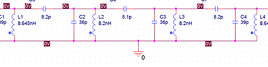# SPICE PARAMETERS

Spice Parameters are used in simulation software. The most used software is LTspice, a SPICE-based analog electronic circuit simulator computer software, produced by semiconductor manufacturer Analog Devices. It is the most widely distributed and used SPICE software in the industry. The "light" version from Linear Technology is freeware, but already this is extremely powerful. Their strategy is simply, the company provides free spice parameters for their integrated circuits. But there is no limitation and nothing against it, to create your own spice parameters for your own components. Be it, an integrated circuit, a transformer, a transistor, and yes, also tubes. Just anything with wires on it.

Spice is not self explaining. So after installing the program, you can not just get a result without reading some of the internet tutorials first. Further below here, I will give you an idea of how it works, but it can not replace a tutorial.

##### **** EML12B ******************************************

* Created on Wed Jan 29 22:05:04 CET 2020 using tube.model.finder.PaintKIT
.SUBCKT EML_12B 1 2 3 ; P G K ;
+ PARAMS: CCG=13.5P  CGP=12P CCP=8P RGI=2000
+ MU=14.616 EX=1.565 KG1=2250.0 KP=300.0 KVB=500.0 VCT=-0.1 ; Vp_MAX=700.0 Ip_MAX=0.1 Vg_step=3.5
E1 7 0 VALUE={V(1,3)/KP*LOG(1+EXP(KP*(1/MU+(V(2,3)+VCT)/SQRT(KVB+V(1,3)*V(1,3)))))}
RE1 7 0 1G
G1 1 3 VALUE={(PWR(V(7),EX)+PWRS(V(7),EX))/KG1}
RCP 1 3 1G ; TO AVOID FLOATING NODES IN MU-FOLLOWER
C1 2 3 {CCG} ; CATHODE-GRID
C2 2 1 {CGP} ; GRID=PLATE
C3 1 3 {CCP} ; CATHODE-PLATE
D3 5 3 DX ; FOR GRID CURRENT
R1 2 5 {RGI} ; FOR GRID CURRENT
.MODEL DX D(IS=1N RS=1 CJO=10PF TT=1N)
.ENDS

##### **** EML20B ******************************************

* Created on 05/27/2022 10:05 using paint_kit.jar 3.1
* www.dmitrynizh.com/tubeparams_image.htm
* Plate Curves image file: EML20B.jpg
*----------------------------------------------------------------------------------
.SUBCKT TRIODE_EML20B 1 2 3 ; Plate Grid Cathode
+ PARAMS: CCG=3P CGP=1.4P CCP=1.9P RGI=2000
+ MU=19.51 KG1=1740 KP=283.05 KVB=7.905 VCT=-2.653 EX=1.454
* Vp_MAX=700 Ip_MAX=100 Vg_step=2 Vg_start=0 Vg_count=11
* Rp=4000 Vg_ac=55 P_max=18.08 Vg_qui=-48 Vp_qui=300
* X_MIN=45 Y_MIN=11 X_SIZE=915 Y_SIZE=734 FSZ_X=1477 FSZ_Y=825 XYGrid=false
* showLoadLine=n showIp=y isDHT=n isPP=n isAsymPP=n showDissipLimit=y
* showIg1=n gridLevel2=n isInputSnapped=n
* XYProjections=n harmonicPlot=n dissipPlot=n
*----------------------------------------------------------------------------------
E1 7 0 VALUE={V(1,3)/KP*LOG(1+EXP(KP*(1/MU+(VCT+V(2,3))/SQRT(KVB+V(1,3)*V(1,3)))))}
RE1 7 0 1G ; TO AVOID FLOATING NODES
G1 1 3 VALUE={(PWR(V(7),EX)+PWRS(V(7),EX))/KG1}
RCP 1 3 1G ; TO AVOID FLOATING NODES
C1 2 3 {CCG} ; CATHODE-GRID
C2 2 1 {CGP} ; GRID=PLATE
C3 1 3 {CCP} ; CATHODE-PLATE
D3 5 3 DX ; POSITIVE GRID CURRENT
R1 2 5 {RGI} ; POSITIVE GRID CURRENT
.MODEL DX D(IS=1N RS=1 CJO=10PF TT=1N)
.ENDS
*\$

### How does SPICE work?

Spice is a virtual test set up. It begins with the REAL circuit diagram, which however you need to re-draw with LT-Spice, so it becomes compatible with the electrical simulation software. Have you ever payed money for electrical circuit drawing software? Well, LT-Spice draws a circuit too, and is freeware. This is a bit nasty to learn, but you will get that done. Lots of "how to" is found in youtube. Then, once the circuit exists in spice format, you can connect electrical signals to it. These can be chosen from the menu, and connected to the circuit. You can connect a DC supply voltage to the circuit, and connect a sine wave to the input. Load the output with an 8 Ohms resistor, and the amplifier works now as a simulation. WIth virtual voltage probes and current probes, you can measure everywhere the signals. A loudspeaker is not an ideal 8 Ohns resistor, it has some inductance too. So you could even simulate the loudspeaker.

##### How can it simulate a complete amplifier?

Spice is based on a "network and node" system, in which each component used, has "nodes", which are it's connections. Nodes are connected by wires. The program doesn't calculate the overall result, like an engineer would do, who understands the schematic. Spice only looks at each electronic part in the schematic. Like when there is a resistor, there is only the relation between current and voltage. If you know what goes in, you know for sure what comes out. The same applies for a capacitor. Like this, the signals in the whole circuit can be constructed in software. For a tube we only would need to put into a formula, what comes out in response to what goes in. This formula has to correspond to the tube curves. This is of course very difficult to do, but there are programs for that as well. Or, use Spice parameters for a particular tube, if somebody made them before. Even so, with the Spice parameters you can of course also draw the tube chart.

So, the beginning of a simulation is always, to build the schematic in LTspice software. These schematics look good, and they always look the same. You will immediately recognise the "looks" of a schematic, made with LTspice.When you see for instance a Push Pull EL34 amplifier working for the first time in LTspice, it will probably amaze you totally, and inspire you very much. Such an LTspice schematic is on the Duncan Amps site. Also some great freeware like PSU designer is written with spice. However this is a higher level of programming, even such a high level, you do not recognise any more, it is spice. This was done with the payed version, and Duncan created his freeware, PSU designer with it.